Скачать презентацию A Power Point Tutorial to Accompany macroeconomics

e9e0a3bfd752fac598367712fbe1b866.ppt

• Количество слайдов: 23® A Power. Point Tutorial to Accompany macroeconomics, 5 th ed. N. Gregory Mankiw CHAPTER NINE Introduction to Economic Fluctuations Mannig J. Simidian Chapter Nine 1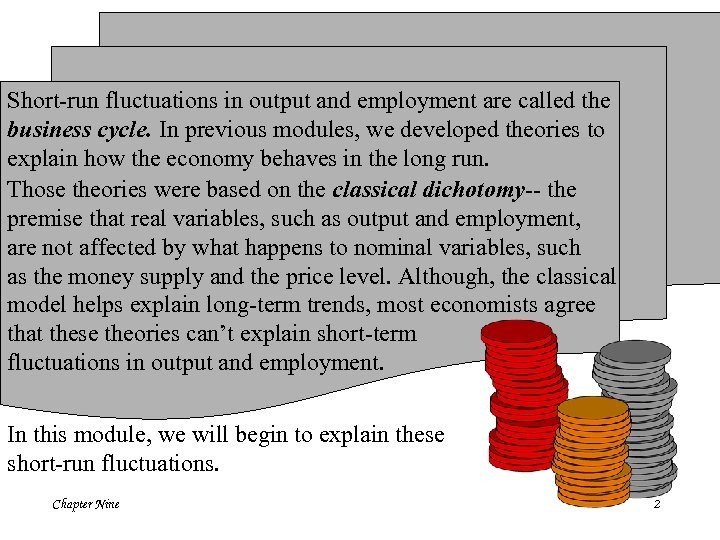Short-run fluctuations in output and employment are called the business cycle. In previous modules, we developed theories to explain how the economy behaves in the long run. Those theories were based on the classical dichotomy-- the premise that real variables, such as output and employment, are not affected by what happens to nominal variables, such as the money supply and the price level. Although, the classical model helps explain long-term trends, most economists agree that these theories can’t explain short-term fluctuations in output and employment. In this module, we will begin to explain these short-run fluctuations. Chapter Nine 2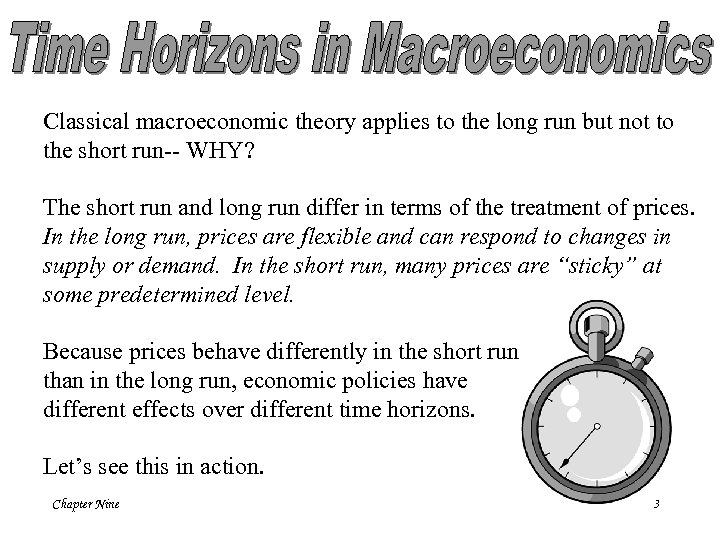Classical macroeconomic theory applies to the long run but not to the short run-- WHY? The short run and long run differ in terms of the treatment of prices. In the long run, prices are flexible and can respond to changes in supply or demand. In the short run, many prices are “sticky” at some predetermined level. Because prices behave differently in the short run than in the long run, economic policies have different effects over different time horizons. Let’s see this in action. Chapter Nine 3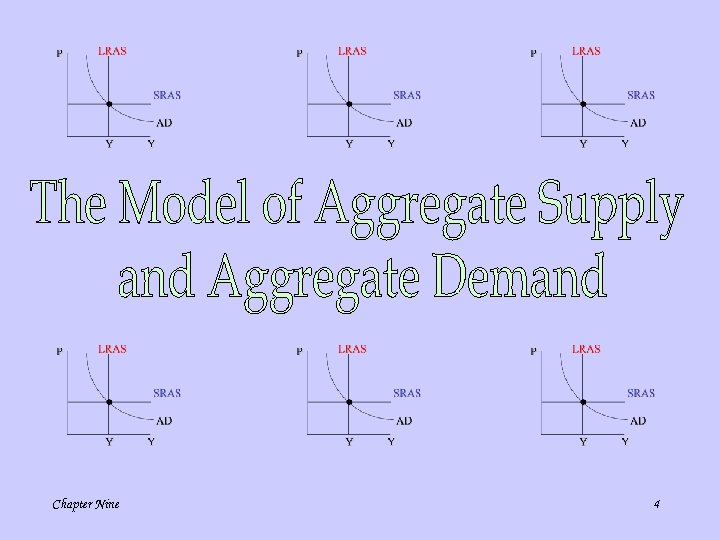Chapter Nine 4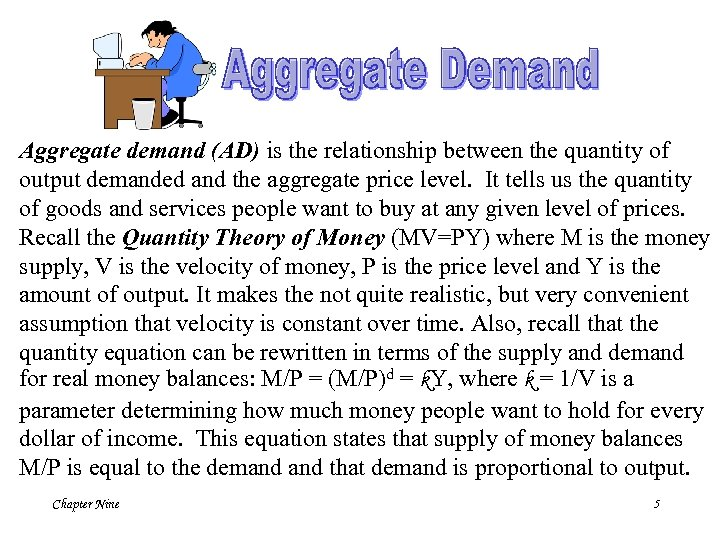Aggregate demand (AD) is the relationship between the quantity of output demanded and the aggregate price level. It tells us the quantity of goods and services people want to buy at any given level of prices. Recall the Quantity Theory of Money (MV=PY) where M is the money supply, V is the velocity of money, P is the price level and Y is the amount of output. It makes the not quite realistic, but very convenient assumption that velocity is constant over time. Also, recall that the quantity equation can be rewritten in terms of the supply and demand for real money balances: M/P = (M/P)d = k. Y, where k = 1/V is a parameter determining how much money people want to hold for every dollar of income. This equation states that supply of money balances M/P is equal to the demand that demand is proportional to output. Chapter Nine 5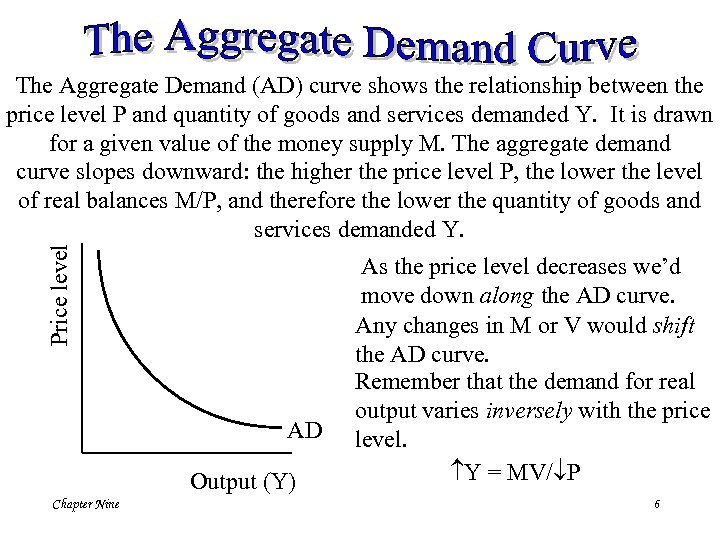Price level The Aggregate Demand (AD) curve shows the relationship between the price level P and quantity of goods and services demanded Y. It is drawn for a given value of the money supply M. The aggregate demand curve slopes downward: the higher the price level P, the lower the level of real balances M/P, and therefore the lower the quantity of goods and services demanded Y. AD Output (Y) Chapter Nine As the price level decreases we’d move down along the AD curve. Any changes in M or V would shift the AD curve. Remember that the demand for real output varies inversely with the price level. Y = MV/ P 6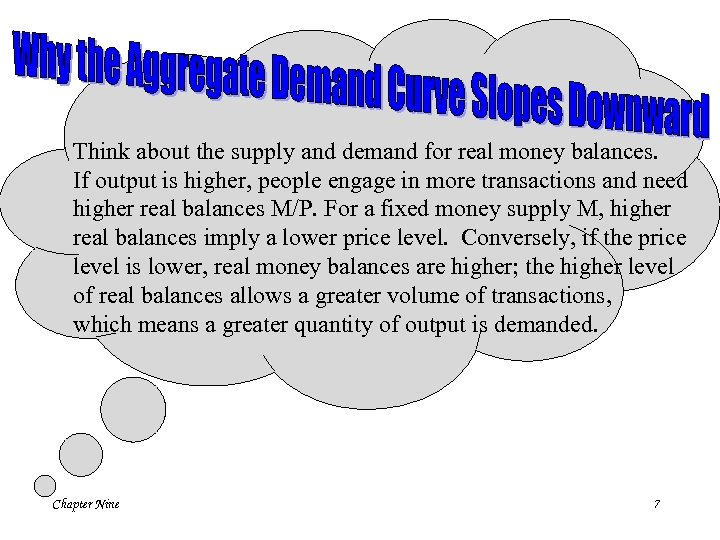Think about the supply and demand for real money balances. If output is higher, people engage in more transactions and need higher real balances M/P. For a fixed money supply M, higher real balances imply a lower price level. Conversely, if the price level is lower, real money balances are higher; the higher level of real balances allows a greater volume of transactions, which means a greater quantity of output is demanded. Chapter Nine 7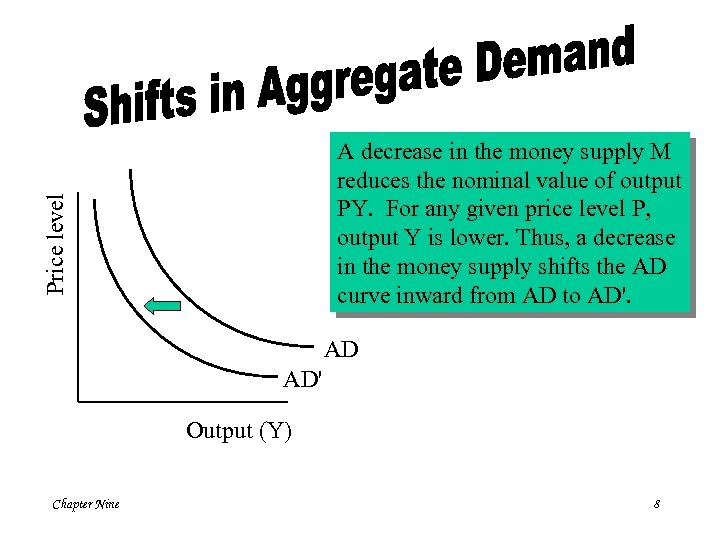Price level A decrease in the money supply M reduces the nominal value of output PY. For any given price level P, output Y is lower. Thus, a decrease in the money supply shifts the AD curve inward from AD to AD'. AD AD' Output (Y) Chapter Nine 8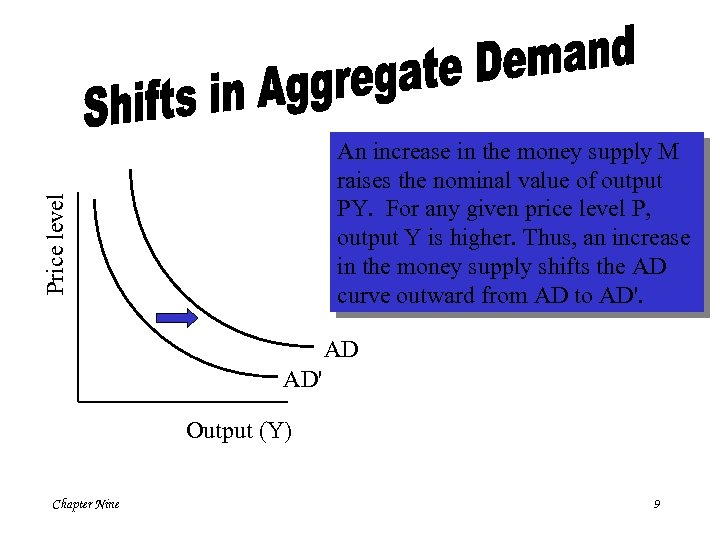Price level An increase in the money supply M raises the nominal value of output PY. For any given price level P, output Y is higher. Thus, an increase in the money supply shifts the AD curve outward from AD to AD'. AD AD' Output (Y) Chapter Nine 9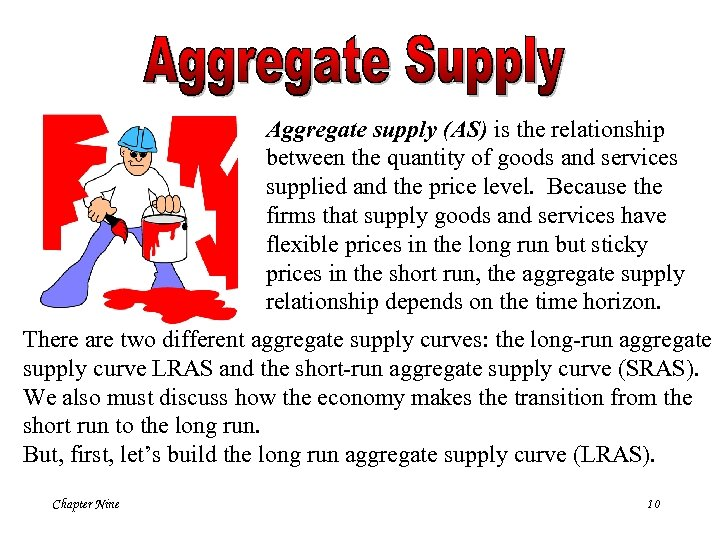Aggregate supply (AS) is the relationship between the quantity of goods and services supplied and the price level. Because the firms that supply goods and services have flexible prices in the long run but sticky prices in the short run, the aggregate supply relationship depends on the time horizon. There are two different aggregate supply curves: the long-run aggregate supply curve LRAS and the short-run aggregate supply curve (SRAS). We also must discuss how the economy makes the transition from the short run to the long run. But, first, let’s build the long run aggregate supply curve (LRAS). Chapter Nine 10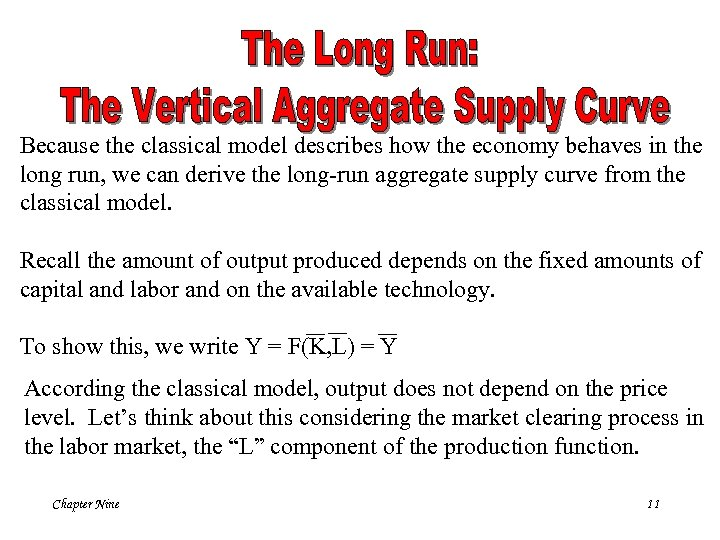Because the classical model describes how the economy behaves in the long run, we can derive the long-run aggregate supply curve from the classical model. Recall the amount of output produced depends on the fixed amounts of capital and labor and on the available technology. To show this, we write Y = F(K, L) = Y According the classical model, output does not depend on the price level. Let’s think about this considering the market clearing process in the labor market, the “L” component of the production function. Chapter Nine 11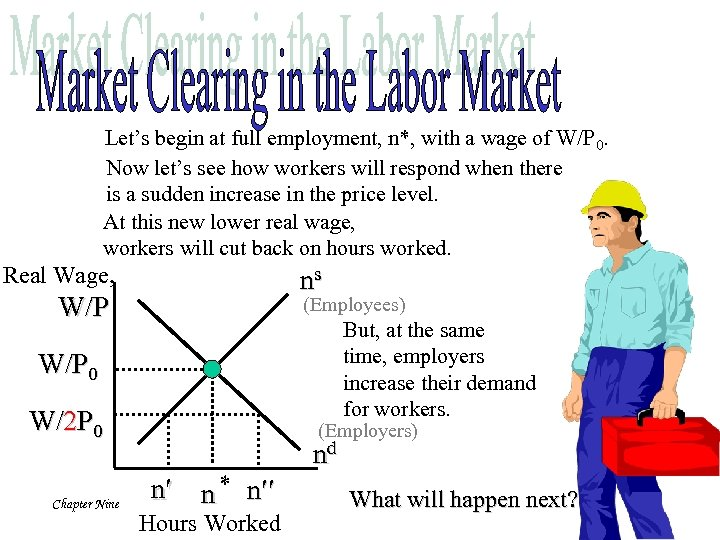Let’s begin at full employment, n*, with a wage of W/P 0. Now let’s see how workers will respond when there is a sudden increase in the price level. At this new lower real wage, workers will cut back on hours worked. Real Wage, ns W/P (Employees) But, at the same time, employers increase their demand for workers. W/P 0 W/2 P 0 Chapter Nine (Employers) nd n n * n Hours Worked What will happen next? 12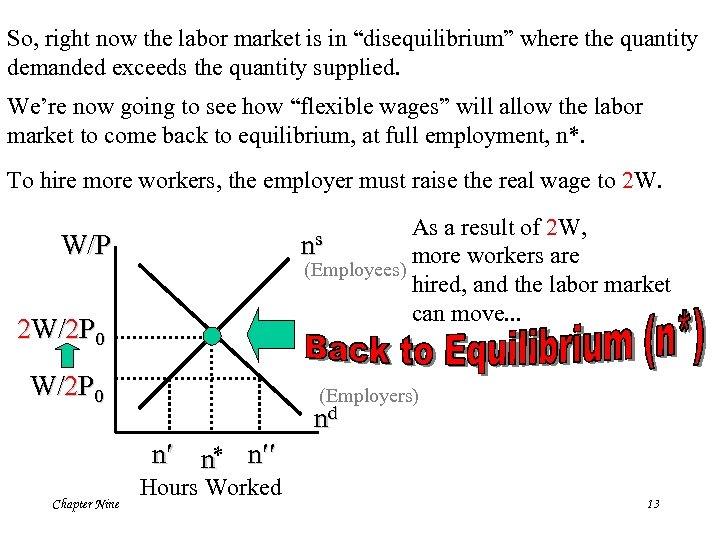So, right now the labor market is in “disequilibrium” where the quantity demanded exceeds the quantity supplied. We’re now going to see how “flexible wages” will allow the labor market to come back to equilibrium, at full employment, n*. To hire more workers, the employer must raise the real wage to 2 W. As a result of 2 W, more workers are (Employees) hired, and the labor market can move. . . W/P ns 2 W/2 P 0 (Employers) nd n n* n Chapter Nine Hours Worked 13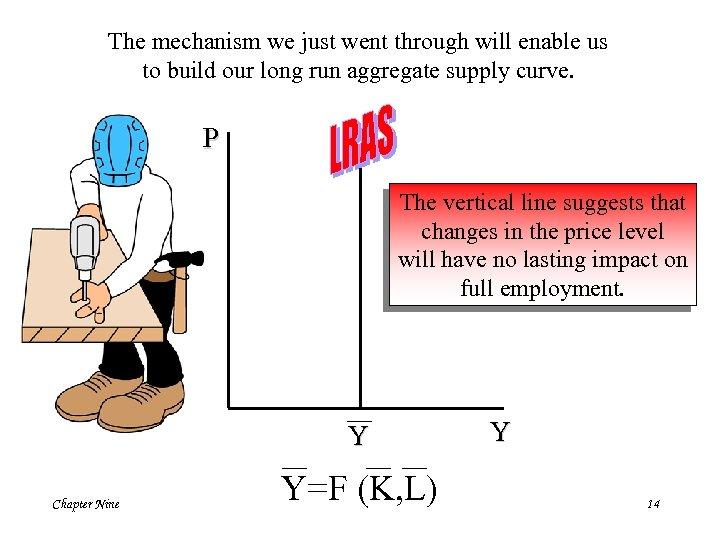The mechanism we just went through will enable us to build our long run aggregate supply curve. P The vertical line suggests that changes in the price level will have no lasting impact on full employment. Y Chapter Nine Y=F (K, L) Y 14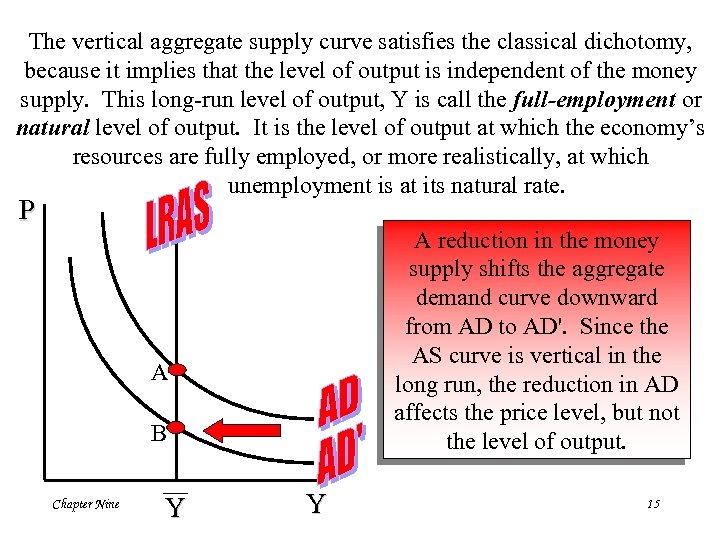The vertical aggregate supply curve satisfies the classical dichotomy, because it implies that the level of output is independent of the money supply. This long-run level of output, Y is call the full-employment or natural level of output. It is the level of output at which the economy’s resources are fully employed, or more realistically, at which unemployment is at its natural rate. P A reduction in the money supply shifts the aggregate demand curve downward from AD to AD'. Since the AS curve is vertical in the long run, the reduction in AD affects the price level, but not the level of output. A B Chapter Nine Y Y 15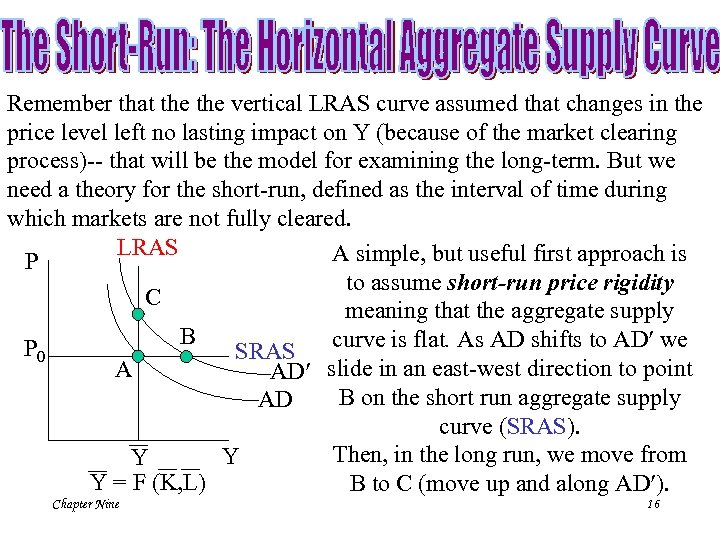Remember that the vertical LRAS curve assumed that changes in the price level left no lasting impact on Y (because of the market clearing process)-- that will be the model for examining the long-term. But we need a theory for the short-run, defined as the interval of time during which markets are not fully cleared. LRAS A simple, but useful first approach is P to assume short-run price rigidity C meaning that the aggregate supply B curve is flat. As AD shifts to AD we P 0 SRAS A AD slide in an east-west direction to point B on the short run aggregate supply AD curve (SRAS). Then, in the long run, we move from Y Y Y = F (K, L) B to C (move up and along AD ). Chapter Nine 16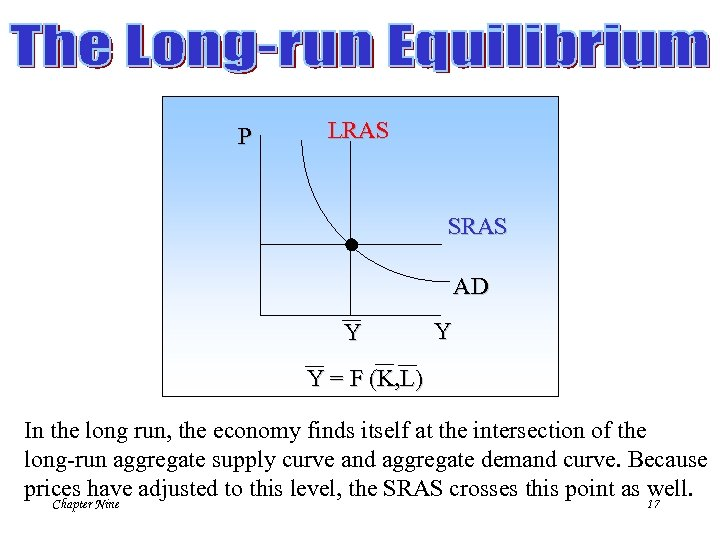P LRAS SRAS AD Y Y Y = F (K, L) In the long run, the economy finds itself at the intersection of the long-run aggregate supply curve and aggregate demand curve. Because prices have adjusted to this level, the SRAS crosses this point as well. Chapter Nine 17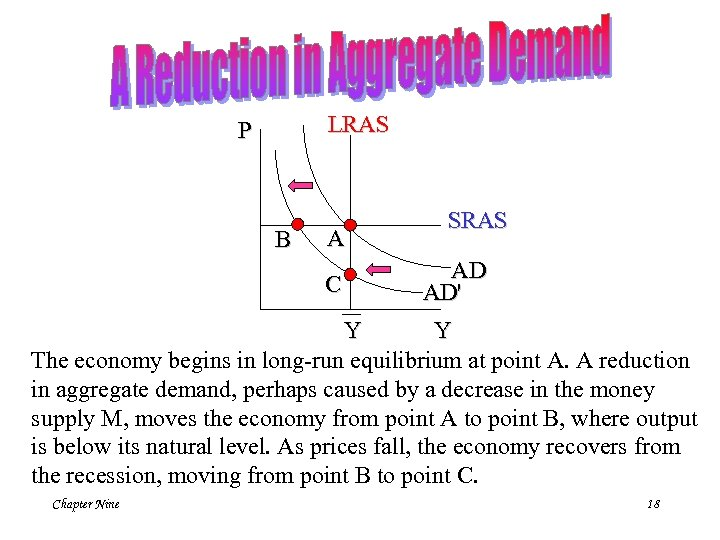LRAS P B A C SRAS AD AD' Y Y The economy begins in long-run equilibrium at point A. A reduction in aggregate demand, perhaps caused by a decrease in the money supply M, moves the economy from point A to point B, where output is below its natural level. As prices fall, the economy recovers from the recession, moving from point B to point C. Chapter Nine 18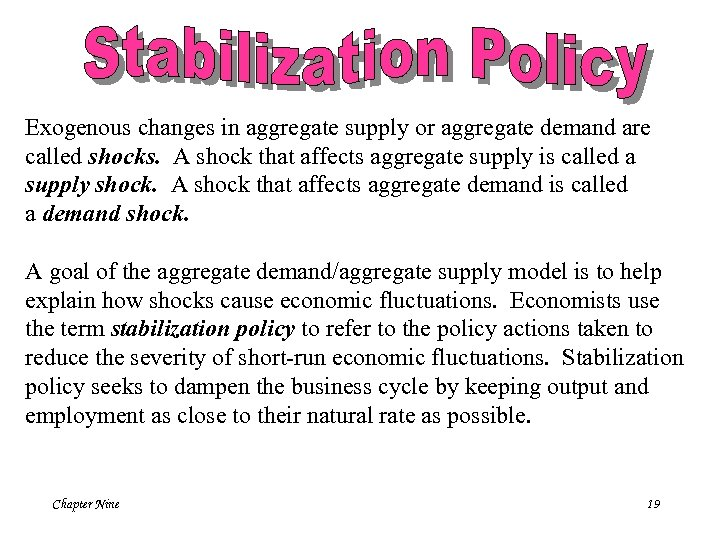Exogenous changes in aggregate supply or aggregate demand are called shocks. A shock that affects aggregate supply is called a supply shock. A shock that affects aggregate demand is called a demand shock. A goal of the aggregate demand/aggregate supply model is to help explain how shocks cause economic fluctuations. Economists use the term stabilization policy to refer to the policy actions taken to reduce the severity of short-run economic fluctuations. Stabilization policy seeks to dampen the business cycle by keeping output and employment as close to their natural rate as possible. Chapter Nine 19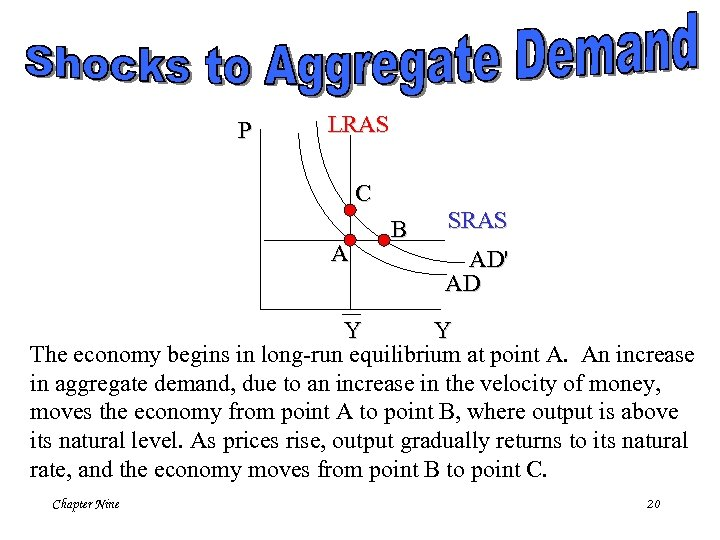P LRAS C A B SRAS AD' AD Y Y The economy begins in long-run equilibrium at point A. An increase in aggregate demand, due to an increase in the velocity of money, moves the economy from point A to point B, where output is above its natural level. As prices rise, output gradually returns to its natural rate, and the economy moves from point B to point C. Chapter Nine 20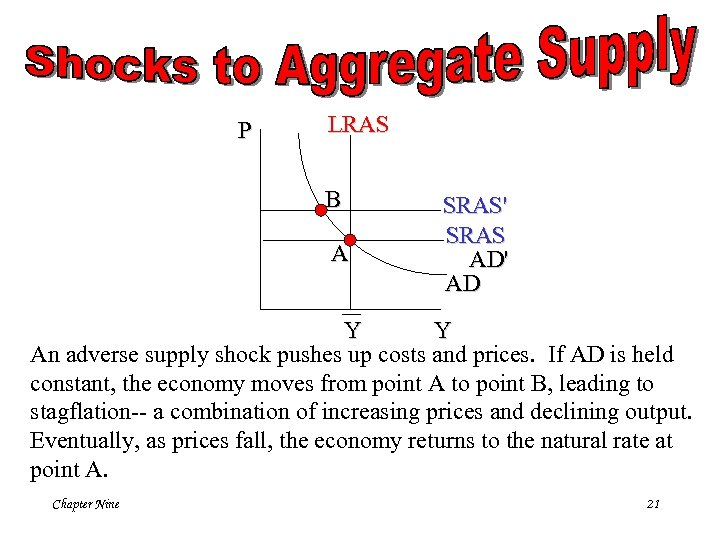P LRAS B A SRAS' SRAS AD' AD Y Y An adverse supply shock pushes up costs and prices. If AD is held constant, the economy moves from point A to point B, leading to stagflation-- a combination of increasing prices and declining output. Eventually, as prices fall, the economy returns to the natural rate at point A. Chapter Nine 21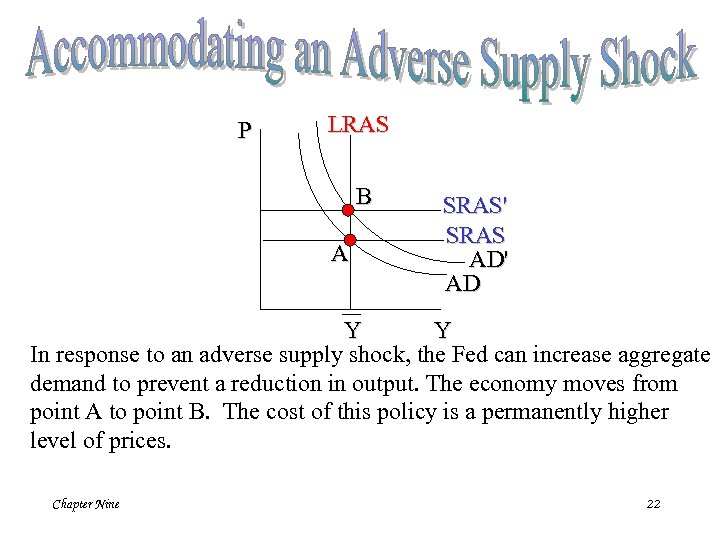P LRAS B A SRAS' SRAS AD' AD Y Y In response to an adverse supply shock, the Fed can increase aggregate demand to prevent a reduction in output. The economy moves from point A to point B. The cost of this policy is a permanently higher level of prices. Chapter Nine 22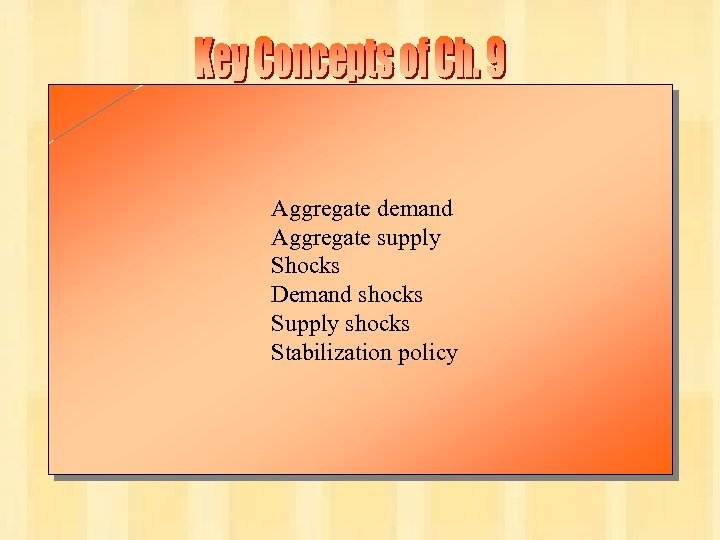Aggregate demand Aggregate supply Shocks Demand shocks Supply shocks Stabilization policy Chapter Nine 23